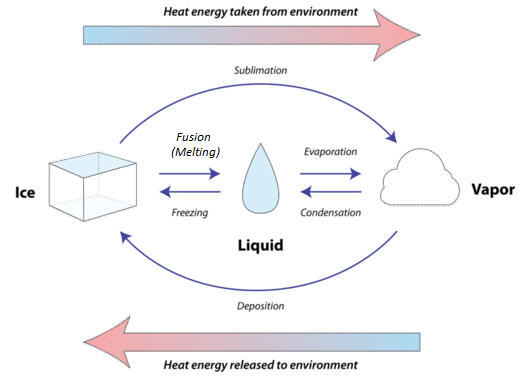# 7.1: States of Matter

$$\newcommand{\vecs}{\overset { \rightharpoonup} {\mathbf{#1}} }$$ $$\newcommand{\vecd}{\overset{-\!-\!\rightharpoonup}{\vphantom{a}\smash {#1}}}$$$$\newcommand{\id}{\mathrm{id}}$$ $$\newcommand{\Span}{\mathrm{span}}$$ $$\newcommand{\kernel}{\mathrm{null}\,}$$ $$\newcommand{\range}{\mathrm{range}\,}$$ $$\newcommand{\RealPart}{\mathrm{Re}}$$ $$\newcommand{\ImaginaryPart}{\mathrm{Im}}$$ $$\newcommand{\Argument}{\mathrm{Arg}}$$ $$\newcommand{\norm}{\| #1 \|}$$ $$\newcommand{\inner}{\langle #1, #2 \rangle}$$ $$\newcommand{\Span}{\mathrm{span}}$$ $$\newcommand{\id}{\mathrm{id}}$$ $$\newcommand{\Span}{\mathrm{span}}$$ $$\newcommand{\kernel}{\mathrm{null}\,}$$ $$\newcommand{\range}{\mathrm{range}\,}$$ $$\newcommand{\RealPart}{\mathrm{Re}}$$ $$\newcommand{\ImaginaryPart}{\mathrm{Im}}$$ $$\newcommand{\Argument}{\mathrm{Arg}}$$ $$\newcommand{\norm}{\| #1 \|}$$ $$\newcommand{\inner}{\langle #1, #2 \rangle}$$ $$\newcommand{\Span}{\mathrm{span}}$$

Learning Outcomes

• List the three states of matter and give examples of each.
• Describe the properties of each state of matter.
• Identify and describe each type of change in state.
• Recognize that boiling and melting points vary with each substance.
• Recognize that a substance's boiling point depends on the pressure.

## States of Matter

Another way that we can describe the properties of matter is the state (also called phase). The amount of energy in molecules of matter determines the state of matter. Matter can exist in one of several different states, including a gas, liquid, or solid state. These different states of matter have different properties, which are illustrated in the figure below.

• A gas is a state of matter in which atoms or molecules have enough energy to move freely. The molecules come into contact with one another only when they randomly collide. Forces between atoms or molecules are not strong enough to hold them together.
• A liquid is a state of matter in which atoms or molecules are constantly in contact but have enough energy to keep changing positions relative to one another. Forces between atoms or molecules are strong enough to keep the molecules relatively close together but not strong enough to prevent them from moving past one another.
• A solid is a state of matter in which atoms or molecules do not have enough energy to move. They are constantly in contact and in fixed positions relative to one another. Forces between atoms or molecules are strong enough to keep the molecules together and to prevent them from moving past one another.Figure $$\PageIndex{1}$$: States of Matter. All three containers contain a substance with the same mass, but the substances are in different states. In the left-hand container, the substance is a gas, which has spread to fill its container. It takes both the shape and volume of the container. In the middle container, the substance is a liquid, which has spread to take the shape of its container but not the volume. In the right-hand container, the substance is a solid, which takes neither the shape nor the volume of its container.

## What Determines a Substance's State?

Which state a substance is in depends partly on temperature and air pressure. For example, at the air pressure found at sea level, water exists as a liquid at temperatures between $$0^\text{o} \text{C}$$ and $$100^\text{o} \text{C}$$. Above $$100^\text{o} \text{C}$$, water exists as a gas (water vapor). Below $$0^\text{o} \text{C}$$, water exists as a solid (ice). Different substances have a different range of temperatures at which they exist in each state. For example, oxygen is a gas above $$-183^\text{o} \text{C}$$, but iron is a gas only above $$2861^\text{o} \text{C}$$. These differences explain why some substances are always solids at normal Earth temperatures, whereas others are always gases or liquids.

Water can take many forms. At low temperatures (below $$0^\text{o} \text{C}$$), it is a solid. It is a liquid between $$0^\text{o} \text{C}$$ and $$100^\text{o} \text{C}$$. While at temperatures above $$100^\text{o} \text{C}$$, water is a gas (steam). The state the water is in depends upon the temperature. Each state (solid, liquid, and gas) has its own unique set of physical properties.

Table $$\PageIndex{1}$$: Characteristics of each Phase of Matter
Gas Liquid Solid

Shape

no definite shape (takes the shape of its container)

no definite shape (takes the shape of its container)

definite shape (rigid)

Volume

particles move in random motion with little or no attraction to each other

has definite volume

definite volume

Mobility

particles move in random motion with little or no attraction to each other

particles are free to move over each other, but are still attracted to each other

particles vibrate around fixed axes

Compressibility

highly compressible

weakly compressible

weakly compressible

Technically speaking a fourth state of matter called plasma exists, but it does not naturally occur on earth, so we will omit it from our study here.

## Changing States

Matter constantly goes through cycles that involve changing states (also called change phases). Water and all the elements important to organisms, including carbon and nitrogen, are constantly recycled on Earth. As matter moves through its cycles, it changes state repeatedly. For example, in the water cycle, water repeatedly changes from a gas to a liquid or solid and back to a gas again. How does this happen?

Adding energy to matter gives its atoms or molecules the ability to resist some of the forces holding them together. For example, heating ice to its melting point gives its molecules enough energy to move. The ice melts and becomes liquid water. Similarly, heating liquid water to its boiling point gives its molecules enough energy to pull apart from one another so they no longer have contact. The liquid water vaporizes and becomes water vapor.Figure $$\PageIndex{2}$$: Matter can move between any two states (or phases) of matter depending on the pressure and temperature conditions. Although the figure shows the states for water, these names apply to all substances. Use of the word fusion for melting is based on its Latin roots.

The temperature of the melting and boiling points depend on the identity of the substance and the atmospheric pressure. Each substance has its own boiling and melting points that depend on the properties of the substance, including the strength of its intermolecular forces. As an example, the values for water are given in Table $$\PageIndex{1}$$. Note how the boiling point of water varies greatly with pressure.

Table $$\PageIndex{1}$$: Boiling point of water as a function of pressure
Altitude $$\left( \text{ft} \right)$$ Pressure $$\left( \text{atm} \right)$$ Boiling Point $$\left( ^\text{o} \text{C} \right)$$
-500 1.05 100.5
0 1.00 100
4000 0.892 96
7000 0.797 93